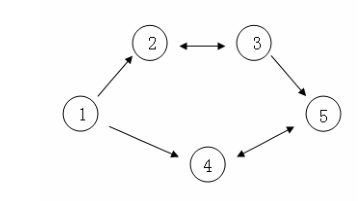[NOIP2009] 最优贸易 luogu P1073 - dfs

题目描述 Description

C

C输入样例 Sample Input：

5 5
4 3 5 6 1
1 2 1
1 4 1
2 3 2 3 5 1 4 5 2

5

题解 Solution：

dfs一遍，更新从1号节点到当前节点的最小价格，并用最大价格减最小价格更新答案，不能更新时结束。这样就可以处理环的情况，复杂度O(n)。

代码 Code：

1 #include<algorithm>
2 #include<iostream>
3 #include<cstring>
4 #include<cstdio>
5 #include<bitset>
6 #include<vector>
7 #include<cmath>
8 #include<ctime>
9 #include<queue>
10 #include<stack>
11 #include<map>
12 #include<set>
13 #define N 100010
14 #define M 1000010
15 #define ll long long
16 #define INF 0x3f3f3f3f
17 using namespace std;
18 int n, m, cnt, ans = -INF;
19 int val[N], mnv[N], f[N];
20 int to[M], nx[M], fir[N];
21 int read() {
22     int x = 0, f = 1;    char ch = getchar();
23     while(ch <  '0' || ch >  '9'){if(ch == '-')f = -1;ch = getchar();}
24     while(ch >= '0' && ch <= '9'){x = x*10 + ch - '0';ch = getchar();}
25     return x*f;
26 }
27 void ins(int u,int v) {
28     to[++cnt] = v, nx[cnt] = fir[u], fir[u] = cnt;
29 }
30 void dfs(int u, int mval, int fa) {
31     int frog = 1;
32     if(val[u] < mval) mval = val[u];
33     if(mnv[u] > mval) mnv[u] = mval, frog = 0;
34     int temp = max(val[u] - mnv[u], f[fa]);
35     if(f[u] < temp) f[u] = temp, frog = 0;
36     if(frog == 1) return;
37     for(int i = fir[u]; i; i = nx[i])
38         dfs(to[i], mval, u);
39 }
40 int main() {
41     n = read(); m = read();
42     for(int i = 1; i <= n; ++i) val[i] = read(), mnv[i] = INF;
43     for(int i = 1; i <= m; ++i) {
44         int x, y, o;
45         x = read(); y = read(); o = read();
46         ins(x, y); if(o == 2) ins(y, x);
47     }
48     dfs(1, INF, 0);
49     cout<<f[n]<<endl;
50     return 0;
51 }

posted @ 2018-05-26 20:43 zhang9302002 阅读(...) 评论(...) 编辑 收藏